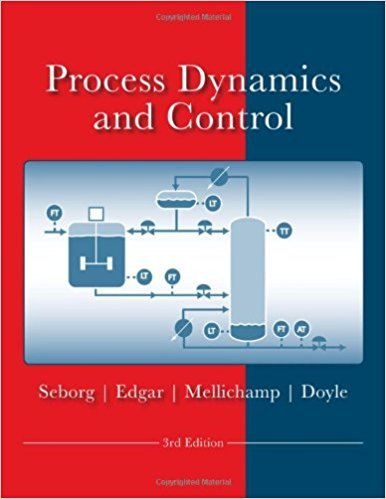×
Get Full Access to Process Dynamics And Control - 3 Edition - Chapter 18 - Problem 18.19
Get Full Access to Process Dynamics And Control - 3 Edition - Chapter 18 - Problem 18.19

×

# A schematic diagram for a pH neutralization process isISBN: 9780470128671 148

## Solution for problem 18.19 Chapter 18

Process Dynamics and Control | 3rd Edition

• Textbook Solutions
• 2901 Step-by-step solutions solved by professors and subject experts
• Get 24/7 help from StudySoup virtual teaching assistantsProcess Dynamics and Control | 3rd Edition

4 5 1 417 Reviews
16
5
Problem 18.19

A schematic diagram for a pH neutralization process is shown in Fig. E18.19. The transfer function matrix and relative gain array are also shown. (a) Suppose that a multiloop control system consisting of four PID controllers is to be designed. Recommend a pairing of controlled and manipulated variables. Briefly justify your recommendation based on steady-state, dynamic, and physical considerations. (b) Suppose that only pH2 and h2 are to be controlled using Q4 and Q6 as the manipulated variables (Q1 and Q3 are held constant). (i) What is the RGA for this 2 X 2 control problem? (ii) What pairing of controlled and manipulated variables do you recommend? (Justify your answer.) hl 0.43 e-D.Ss 4.32s+ 1 pHl -D.33 e -l.Os 2.56 s+ 1 hz 0.22 e-Lls 5.52s+ 1 -D.22 e -l.Ss pHz 3.24 s+ 1 0.43 e-D.ls 0.23 e-l.Os 0.22 e-D.Ss 3.10 s + 1 5.24 s+ 1 4.42 s + 1 0.32 e-D.Ss -0.20 e -l.Ss 0.20 e-D.Ss 2.58 s + 1 2.82s+ 1 3.30 s + 1 0.23 e-D-3s 0.42 e-D.4s 0.41 e -D.ls 4.49 s + 1 3.32 s + 1 2.07 s+ 1 0.22 e-1.2s -0.32 e-D.Ss 0.32 e-D.4s 2.65 s + 1 2.36s+ 1 2.03 s+ 1 Gp-- Figure E18.20 1 I I I I Ql Q3 Q4 Q

Step-by-Step Solution:

Problem 18.19A schematic diagram for a pH neutralization process is shown in Fig. E18.19. The transferfunction matrix and relative gain array are also shown.(a) Suppose that a multiloop control system consisting of four PID controllers is to be designed.Recommend a pairing of controlled and manipulated variables. Briefly justify yourrecommendation based on steady-state, dynamic, and physical considerations.(b) Suppose that only pH and h are to be controlled using Q and Q as the manipulated 2 2 4 6variables (Q and Q are held constant). 1 3 (i) What is the RGA for this 2 X 2 control problem (ii) What pairing of controlled and manipulated variables do you recommend (Justify your answer.) Step-by-step solution Step 1 of 6 ^(a)Refer to Figure E18.19 in the text book.In the statistic considerations, pair the controlled and manipulated variables so thatcorresponding relative gains are positive and as close to one as possible. The painting for eachtank between controlled and manipulated variables are, h - Q pH - Q ,1 -3 an1pH1 Q2 4 2 6In the dynamic considerations, the some pairing has the smallest time constants for tank 1 andalso dynamically best for tank 2, because these pairing avoids the large ratio of time delay () totime constant ( ).In the physical consideration, the given pairing makes senses because the controlled variablesfor each tank are paired with the inlet flows for that some tank. Because pH is more importantthan tank level, In the pairing h - Q and pH - Q , the first tank has the smaller time delay. It 1 1 1 3provides better pH control.

Step 2 of 6

Step 3 of 6

##### ISBN: 9780470128671

This textbook survival guide was created for the textbook: Process Dynamics and Control, edition: 3. The full step-by-step solution to problem: 18.19 from chapter: 18 was answered by , our top Engineering and Tech solution expert on 11/15/17, 04:03PM. Since the solution to 18.19 from 18 chapter was answered, more than 400 students have viewed the full step-by-step answer. Process Dynamics and Control was written by and is associated to the ISBN: 9780470128671. The answer to “A schematic diagram for a pH neutralization process is shown in Fig. E18.19. The transfer function matrix and relative gain array are also shown. (a) Suppose that a multiloop control system consisting of four PID controllers is to be designed. Recommend a pairing of controlled and manipulated variables. Briefly justify your recommendation based on steady-state, dynamic, and physical considerations. (b) Suppose that only pH2 and h2 are to be controlled using Q4 and Q6 as the manipulated variables (Q1 and Q3 are held constant). (i) What is the RGA for this 2 X 2 control problem? (ii) What pairing of controlled and manipulated variables do you recommend? (Justify your answer.) hl 0.43 e-D.Ss 4.32s+ 1 pHl -D.33 e -l.Os 2.56 s+ 1 hz 0.22 e-Lls 5.52s+ 1 -D.22 e -l.Ss pHz 3.24 s+ 1 0.43 e-D.ls 0.23 e-l.Os 0.22 e-D.Ss 3.10 s + 1 5.24 s+ 1 4.42 s + 1 0.32 e-D.Ss -0.20 e -l.Ss 0.20 e-D.Ss 2.58 s + 1 2.82s+ 1 3.30 s + 1 0.23 e-D-3s 0.42 e-D.4s 0.41 e -D.ls 4.49 s + 1 3.32 s + 1 2.07 s+ 1 0.22 e-1.2s -0.32 e-D.Ss 0.32 e-D.4s 2.65 s + 1 2.36s+ 1 2.03 s+ 1 Gp-- Figure E18.20 1 I I I I Ql Q3 Q4 Q” is broken down into a number of easy to follow steps, and 213 words. This full solution covers the following key subjects: variables, controlled, manipulated, pairing, recommend. This expansive textbook survival guide covers 24 chapters, and 420 solutions.

Unlock Textbook Solution